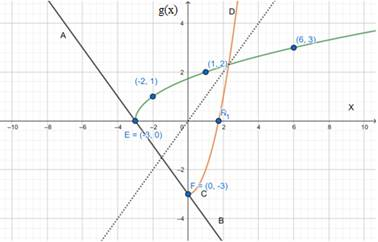# The inverse function of g ( x ) = x + 3 .### Precalculus: Mathematics for Calcu...

6th Edition
Stewart + 5 others
Publisher: Cengage Learning
ISBN: 9780840068071### Precalculus: Mathematics for Calcu...

6th Edition
Stewart + 5 others
Publisher: Cengage Learning
ISBN: 9780840068071

#### Solutions

Chapter 2.7, Problem 73E

a.

To determine

## To find: The inverse function of g(x)=x+3 .

Expert Solution

g1(x)=x23

### Explanation of Solution

Given information: g(x)=x+3

Concept Use: Let, f be a one-to-one function with domain A and range B. Then its inverse function f1 has domain B and range A and is defining by f1(y)=xf(x)=y for any y in B.

Calculation:

First, we replace g(x) with y ,

Then,

g(x)=x+3y=x+3y2=x+3x=y23g1(y)=y23g1(x)=x23

Thus, g1(x)=x23 .

b.

To determine

Expert Solution

### Explanation of Solution

Given information: g(x)=x+3 and g1(x)=x23

Graph:

 x 1 6 −3 −2 13 g(x) 2 3 0 1 4Interpretation:

A point on the graph of g(x) , (3,0) . The dotted line is the graph of y=x , CD is the graph of g1(x) . AB is the perpendicular on the dotted line from the point (3,0) , It intersects the CD line at point F (0,3) .

It is clear that the coordinate of E is reversed from F.

Thus, it means g(x) and g1(x) is the reflection of each other in the line y=x (Dotted line).

### Have a homework question?

Subscribe to bartleby learn! Ask subject matter experts 30 homework questions each month. Plus, you’ll have access to millions of step-by-step textbook answers!